Share

# Area of a Polygon

#### notes

(i) Pentagon :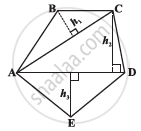By constructing two diagonals AC and AD the pentagon ABCDE is divided into three parts.
So, area ABCDE = area of Triangle ABC + area of Triangle ACD + area of Triangle AED.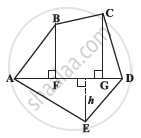By constructing one diagonal AD and two perpendiculars BF and CG on it, pentagon ABCDE is divided into four parts.

So, area of ABCDE
= area of right angled D AFB + area of trapezium BFGC + area of right angled Triangle CGD + area of Triangle AED.

(ii) Hexagon :

⦁   Method 1 :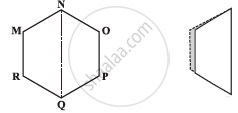Since, it is a hexagon so NQ divides the hexagon into two congruent trapeziums.

Now area of trapezium MNQR = 2 xx {1/2 ("sum of parallel sides") x ("perpendicular distance between them")}

⦁ Method 2 :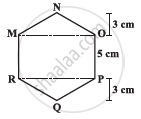Triangle MNO and Triangle RPQ are congruent triangles with altitude.

Area of hexagon MNOPQR= Area of Triangle MNO + Area of Triangle RPQ + Area of Rectangle MOPR

(iii) Octagon: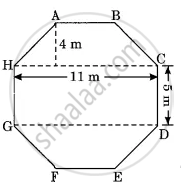Area of Octagonal Surface= Area of trapezium ABCH+ Area of rectangle HCDG + Area of Trapezium GDEF.

S